These are complete themes which control all non-data display. Use theme() if you just need to tweak the display of an existing theme.

## Usage

theme_grey(
base_size = 11,
base_family = "",
base_line_size = base_size/22,
base_rect_size = base_size/22
)

theme_gray(
base_size = 11,
base_family = "",
base_line_size = base_size/22,
base_rect_size = base_size/22
)

theme_bw(
base_size = 11,
base_family = "",
base_line_size = base_size/22,
base_rect_size = base_size/22
)

theme_linedraw(
base_size = 11,
base_family = "",
base_line_size = base_size/22,
base_rect_size = base_size/22
)

theme_light(
base_size = 11,
base_family = "",
base_line_size = base_size/22,
base_rect_size = base_size/22
)

theme_dark(
base_size = 11,
base_family = "",
base_line_size = base_size/22,
base_rect_size = base_size/22
)

theme_minimal(
base_size = 11,
base_family = "",
base_line_size = base_size/22,
base_rect_size = base_size/22
)

theme_classic(
base_size = 11,
base_family = "",
base_line_size = base_size/22,
base_rect_size = base_size/22
)

theme_void(
base_size = 11,
base_family = "",
base_line_size = base_size/22,
base_rect_size = base_size/22
)

theme_test(
base_size = 11,
base_family = "",
base_line_size = base_size/22,
base_rect_size = base_size/22
)

## Arguments

base_size

base font size, given in pts.

base_family

base font family

base_line_size

base size for line elements

base_rect_size

base size for rect elements

## Details

theme_gray()

The signature ggplot2 theme with a grey background and white gridlines, designed to put the data forward yet make comparisons easy.

theme_bw()

The classic dark-on-light ggplot2 theme. May work better for presentations displayed with a projector.

theme_linedraw()

A theme with only black lines of various widths on white backgrounds, reminiscent of a line drawing. Serves a purpose similar to theme_bw(). Note that this theme has some very thin lines (<< 1 pt) which some journals may refuse.

theme_light()

A theme similar to theme_linedraw() but with light grey lines and axes, to direct more attention towards the data.

theme_dark()

The dark cousin of theme_light(), with similar line sizes but a dark background. Useful to make thin coloured lines pop out.

theme_minimal()

A minimalistic theme with no background annotations.

theme_classic()

A classic-looking theme, with x and y axis lines and no gridlines.

theme_void()

A completely empty theme.

theme_test()

A theme for visual unit tests. It should ideally never change except for new features.

## Examples

mtcars2 <- within(mtcars, {
vs <- factor(vs, labels = c("V-shaped", "Straight"))
am <- factor(am, labels = c("Automatic", "Manual"))
cyl  <- factor(cyl)
gear <- factor(gear)
})

p1 <- ggplot(mtcars2) +
geom_point(aes(x = wt, y = mpg, colour = gear)) +
labs(
title = "Fuel economy declines as weight increases",
subtitle = "(1973-74)",
caption = "Data from the 1974 Motor Trend US magazine.",
tag = "Figure 1",
x = "Weight (1000 lbs)",
y = "Fuel economy (mpg)",
colour = "Gears"
)

p1 + theme_gray() # the default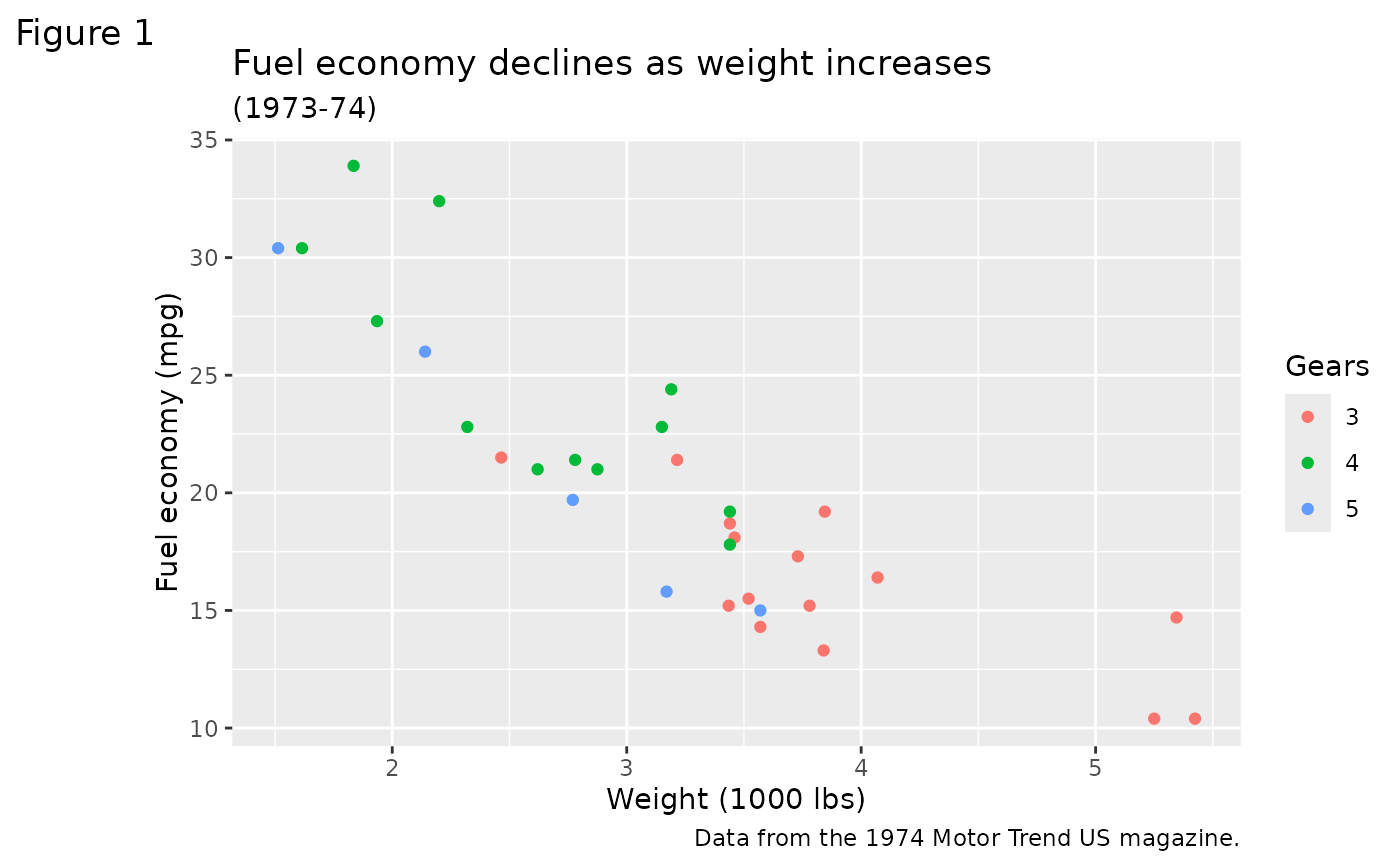p1 + theme_bw()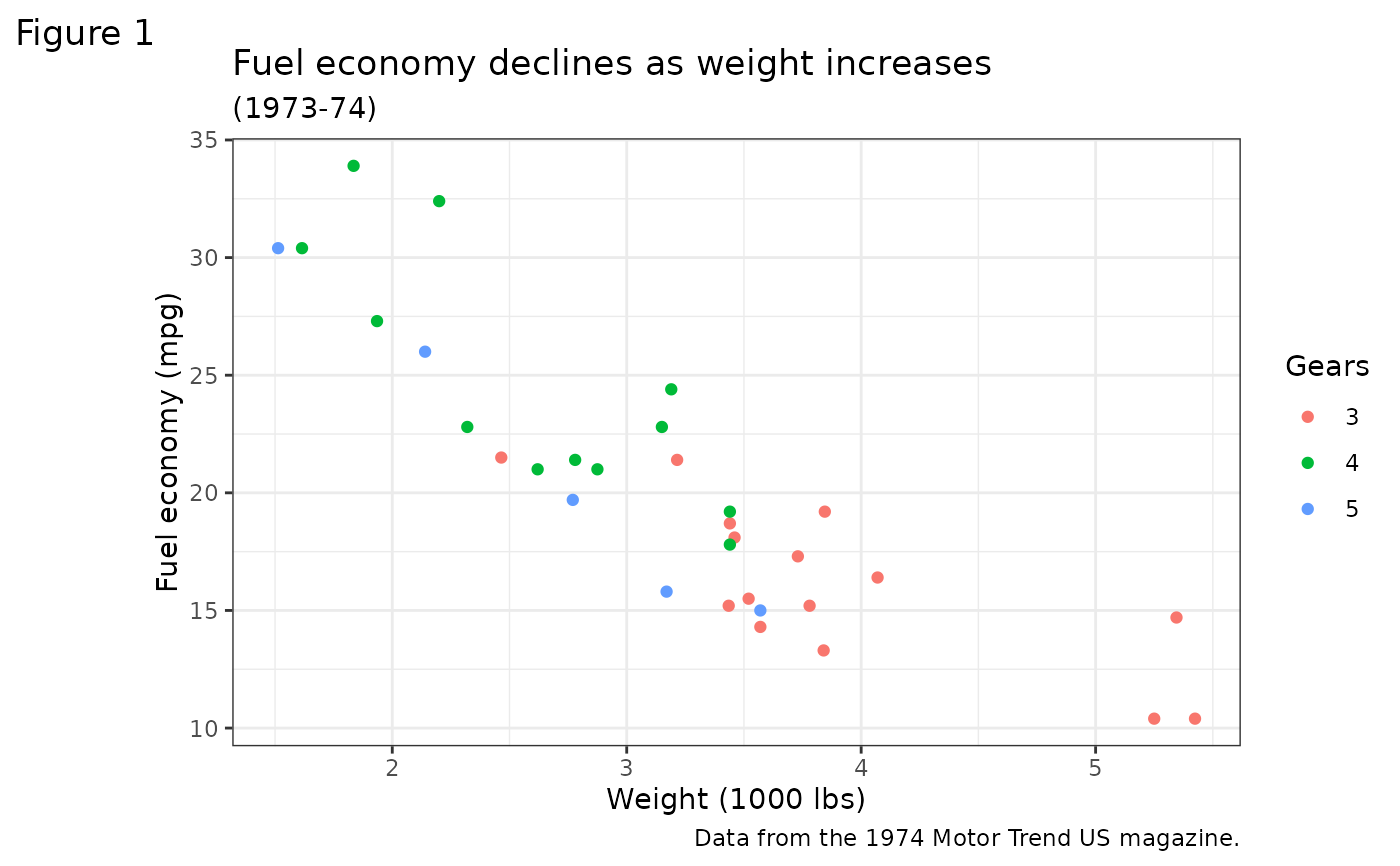p1 + theme_linedraw()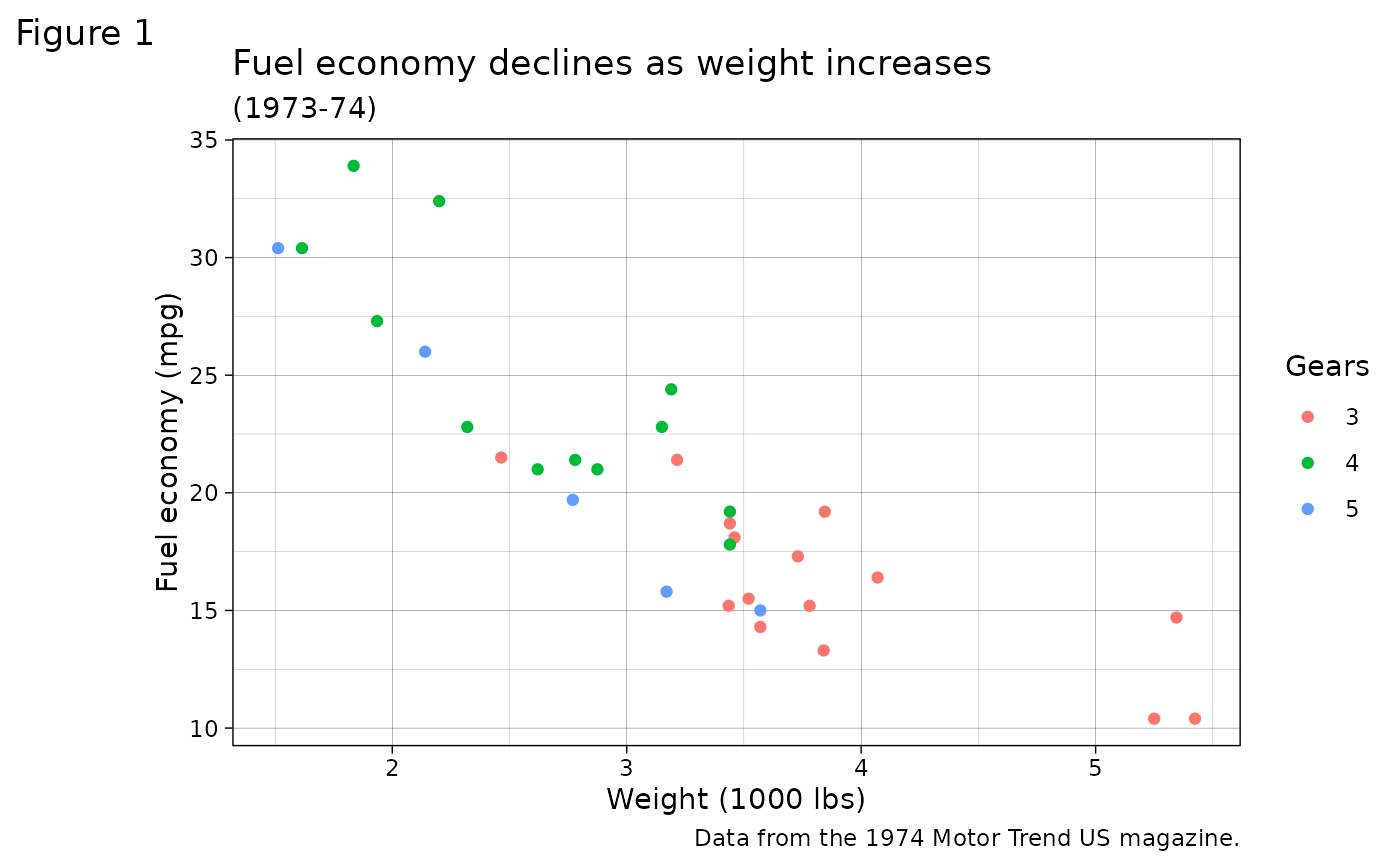p1 + theme_light()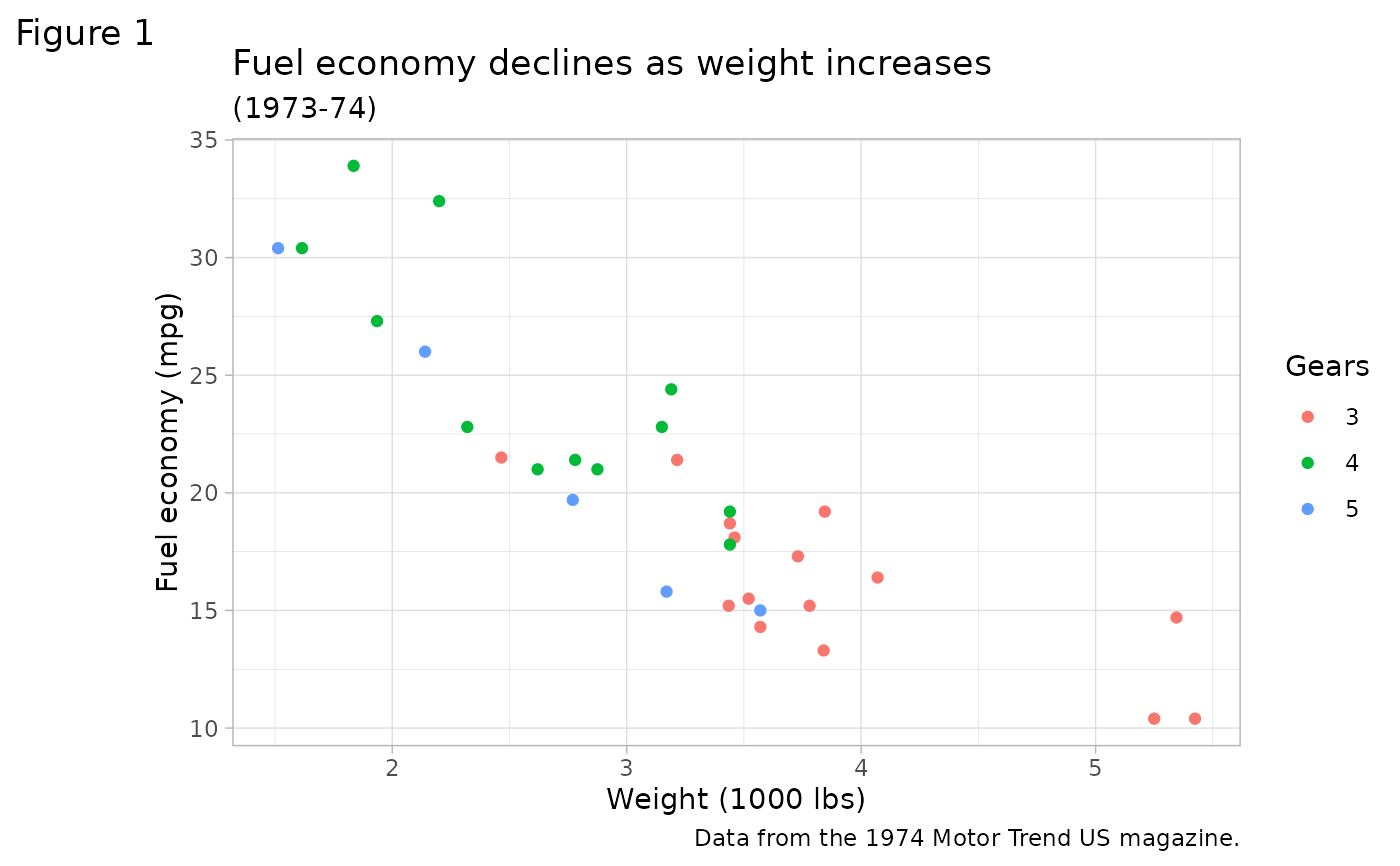p1 + theme_dark()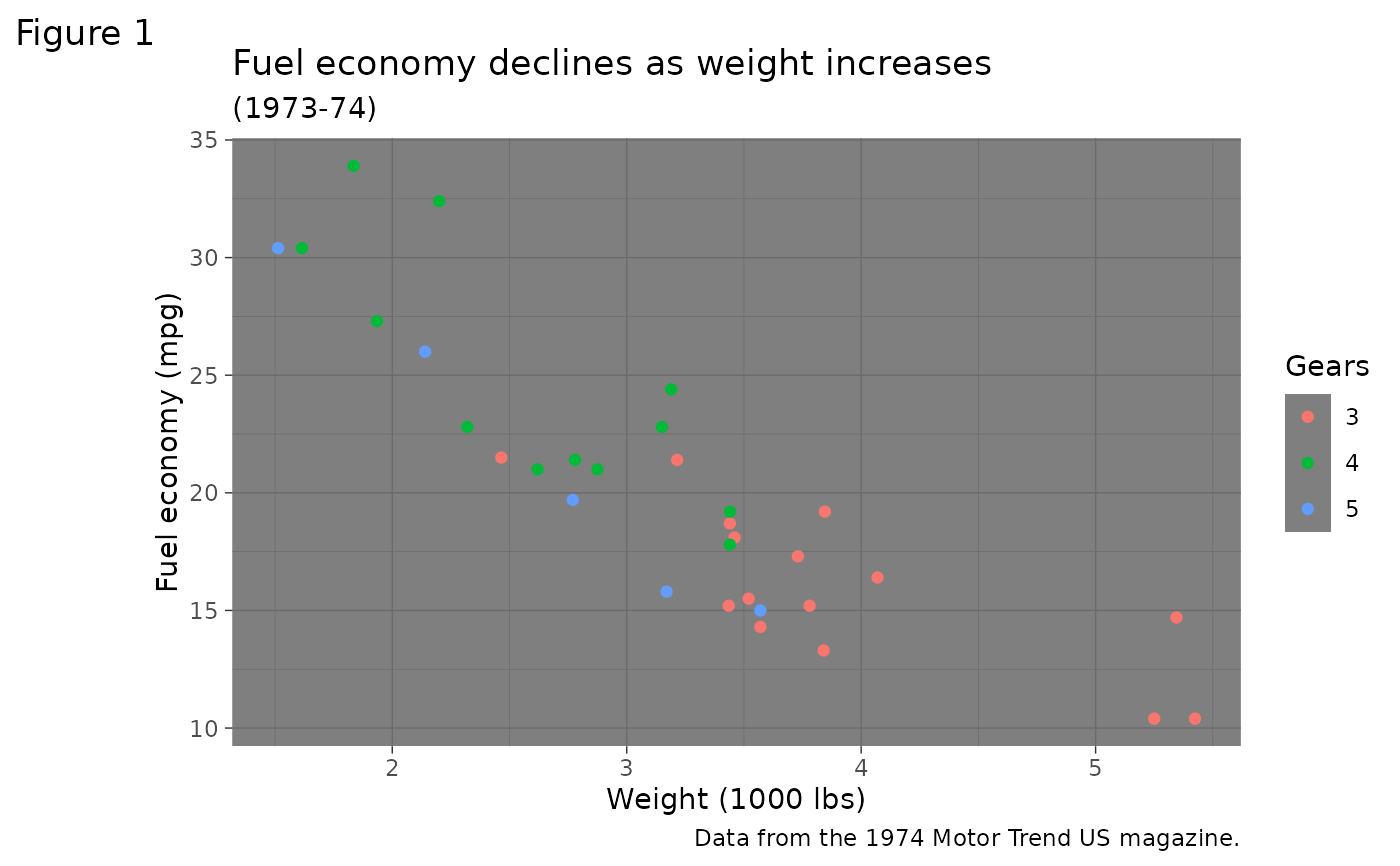p1 + theme_minimal()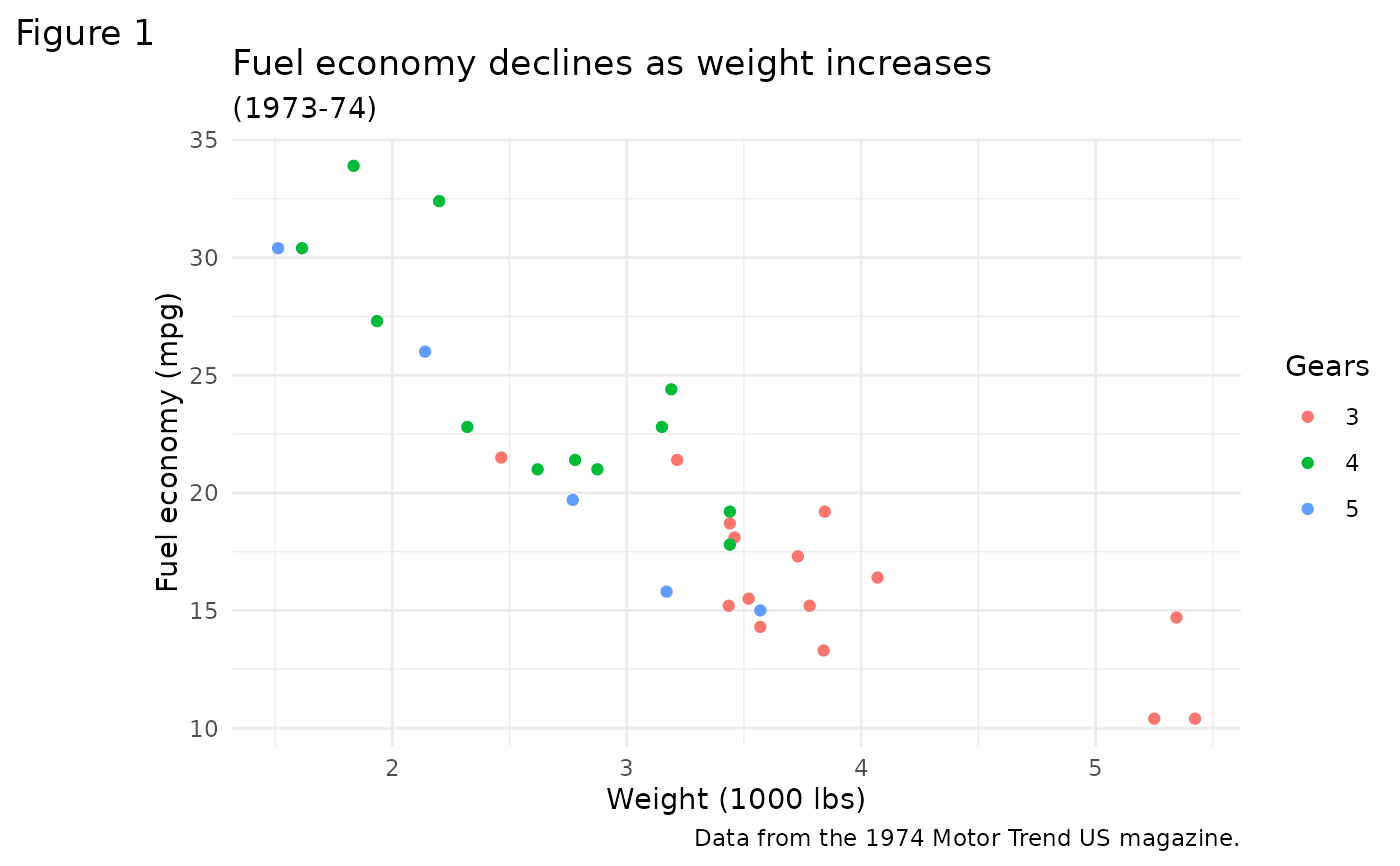p1 + theme_classic()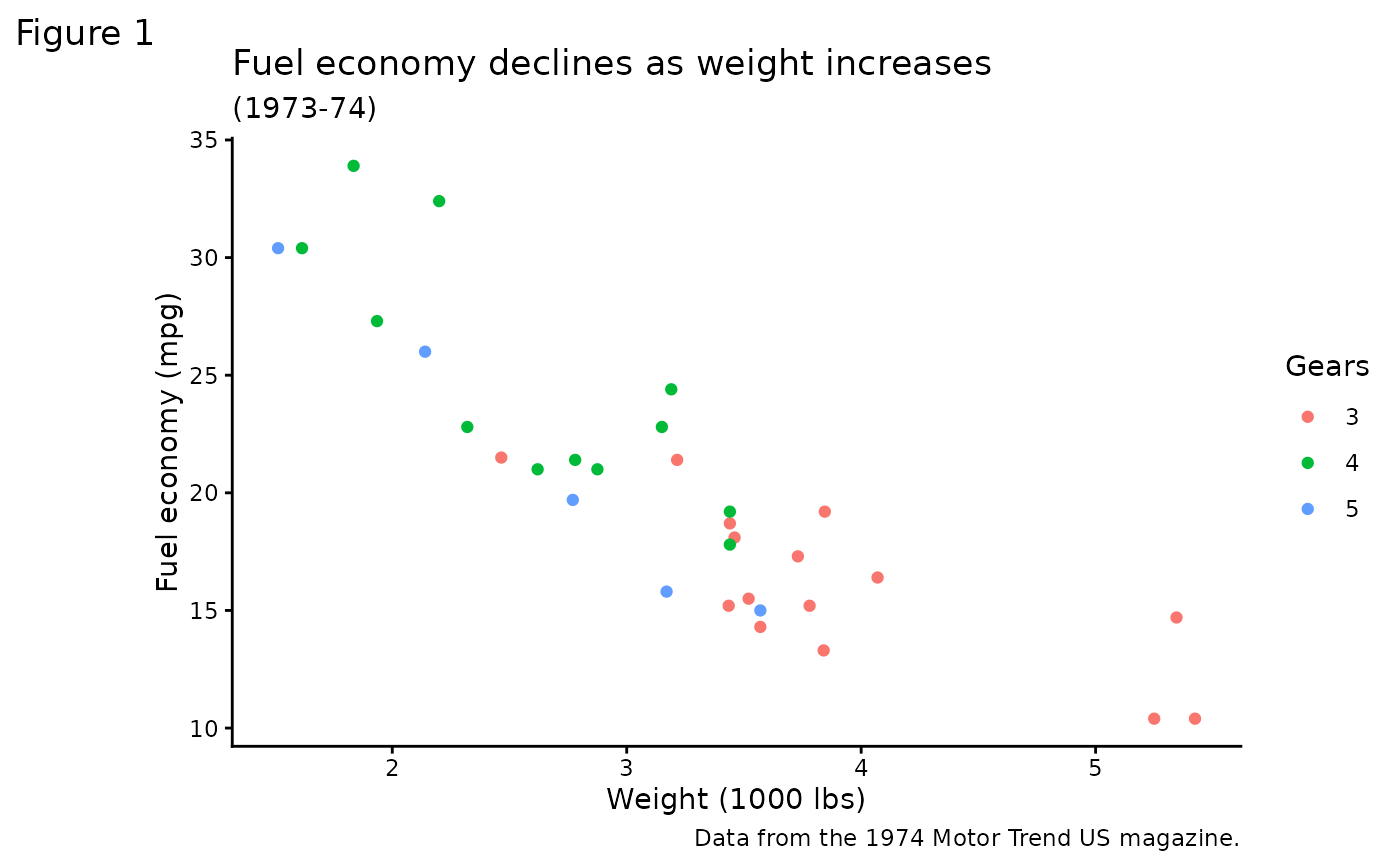p1 + theme_void()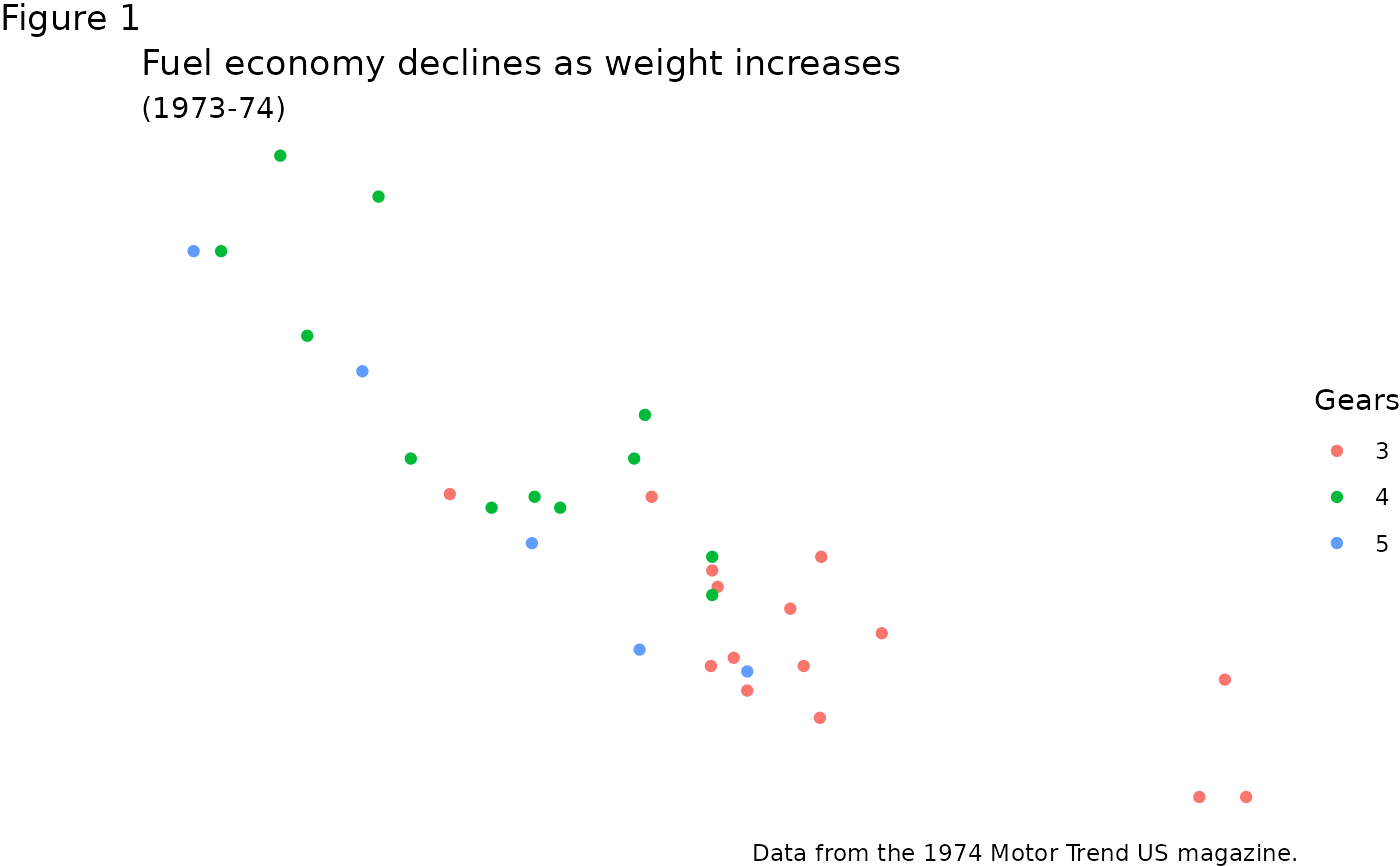# Theme examples with panels
# \donttest{
p2 <- p1 + facet_grid(vs ~ am)

p2 + theme_gray() # the default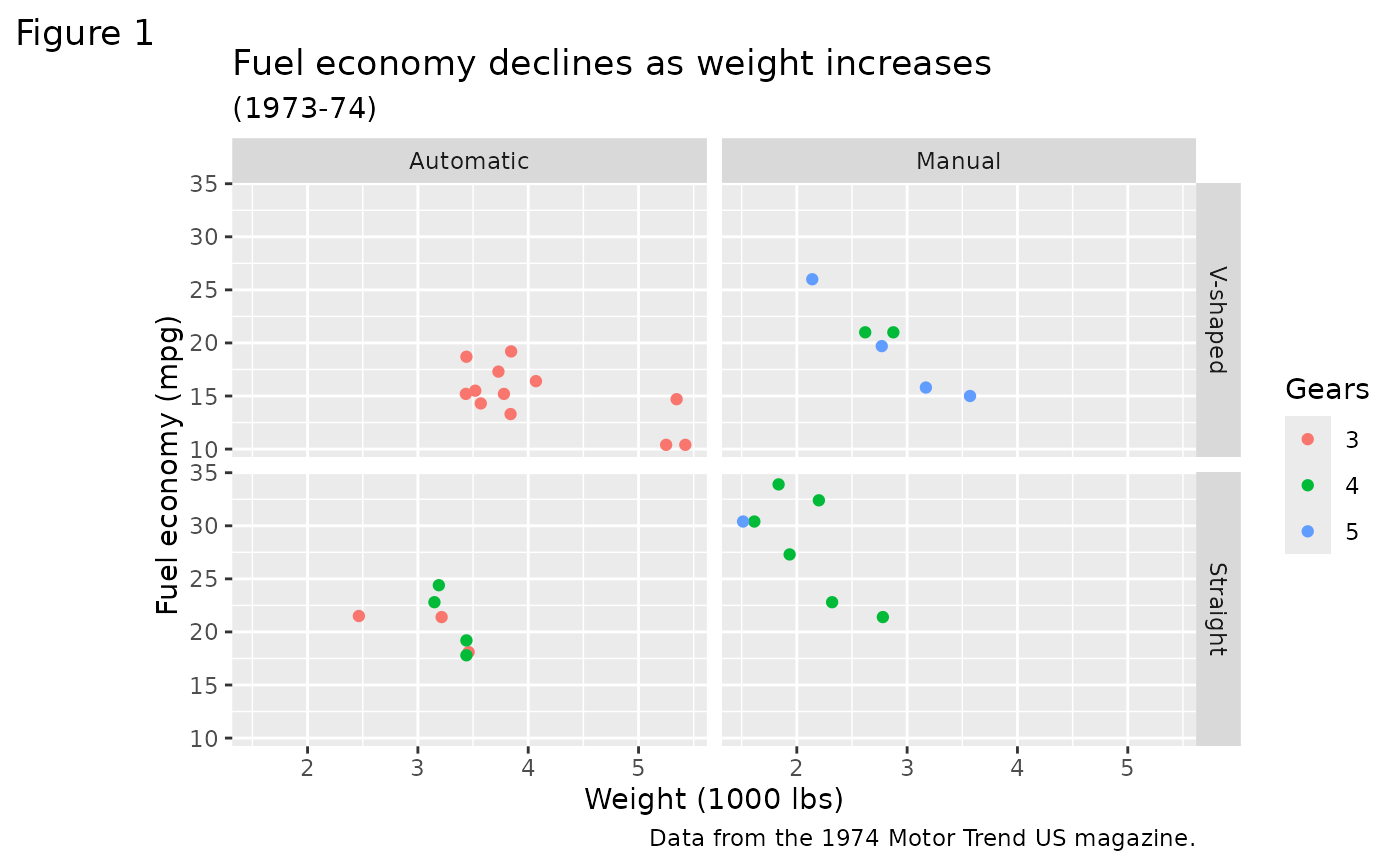p2 + theme_bw()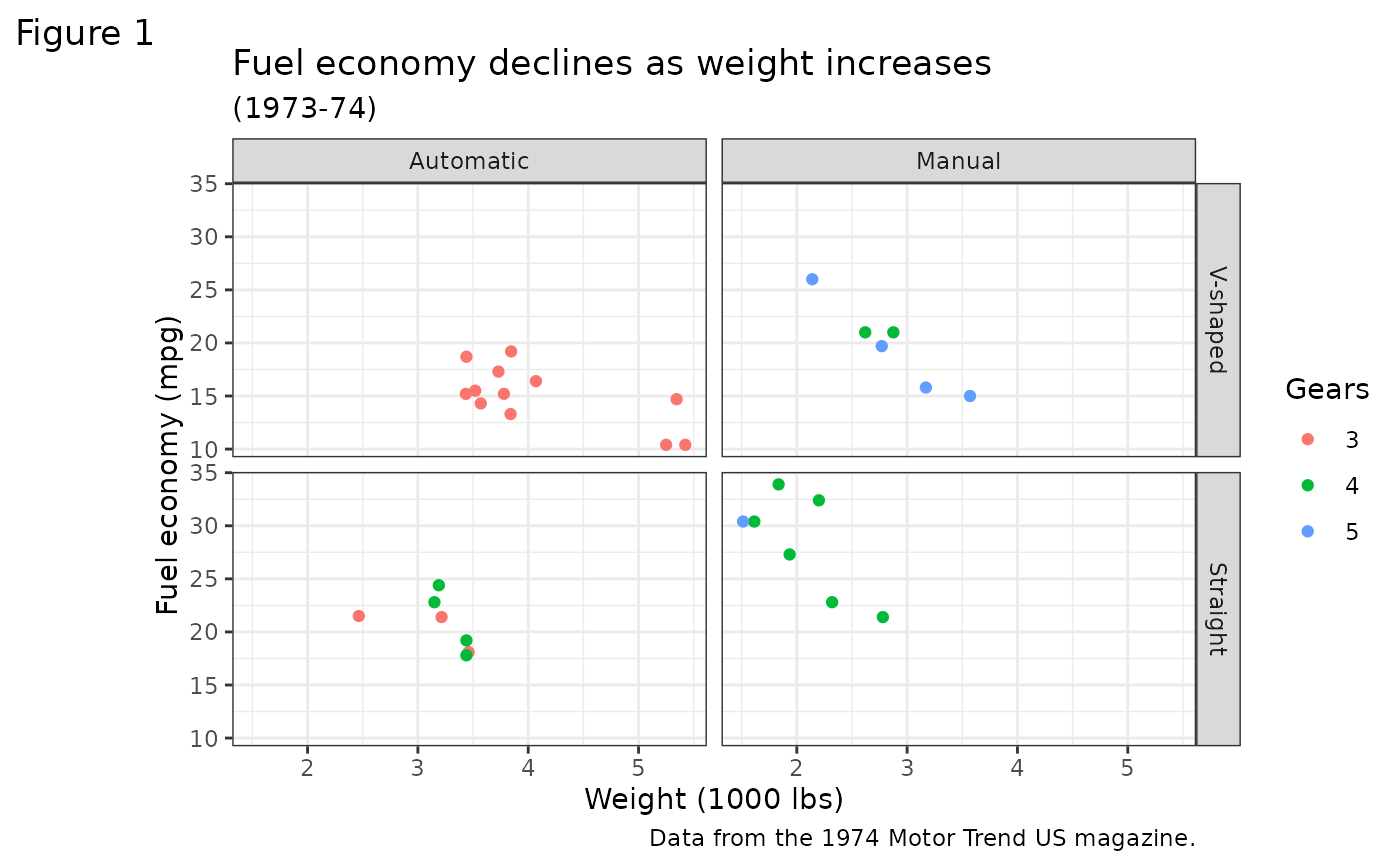p2 + theme_linedraw()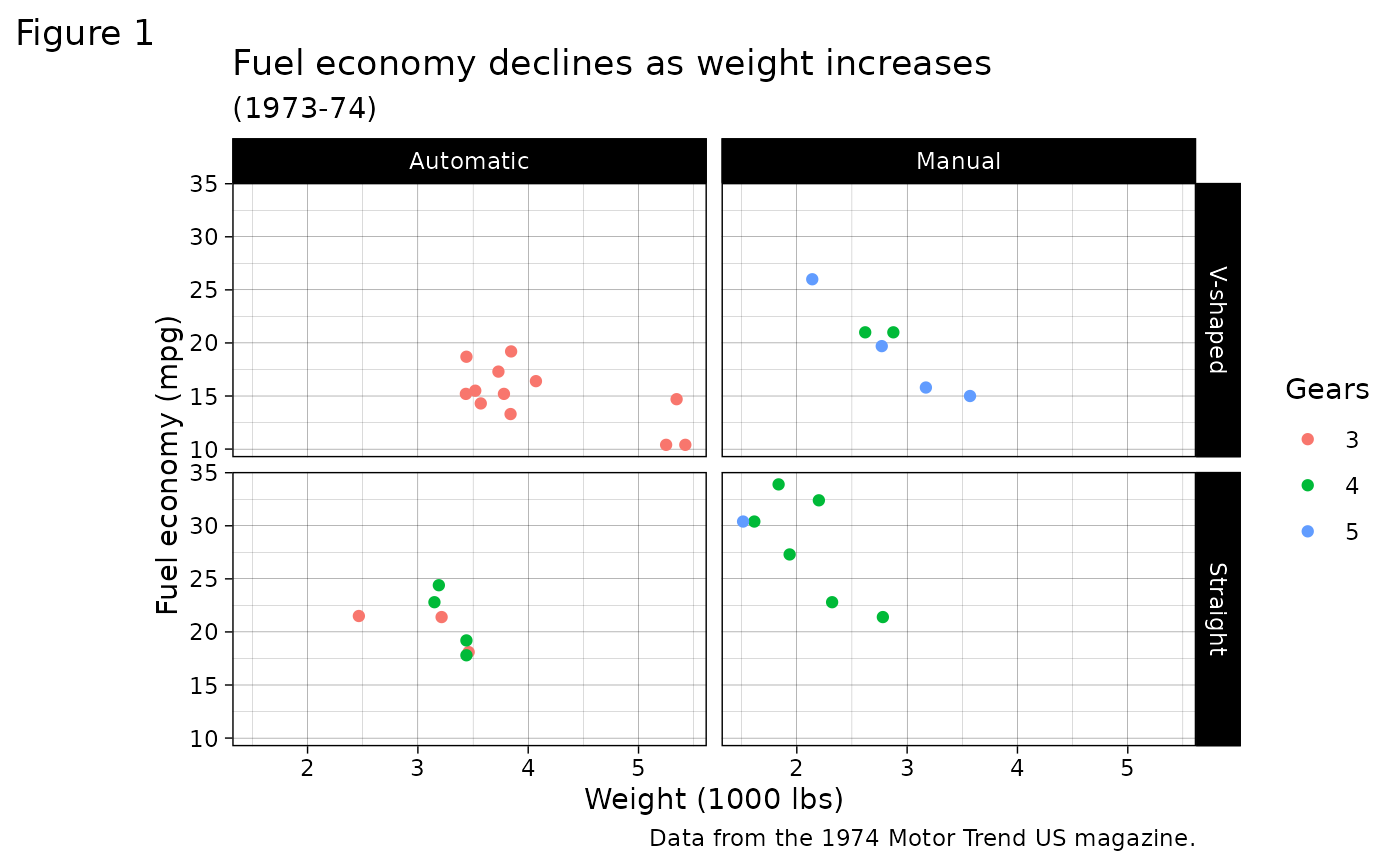p2 + theme_light()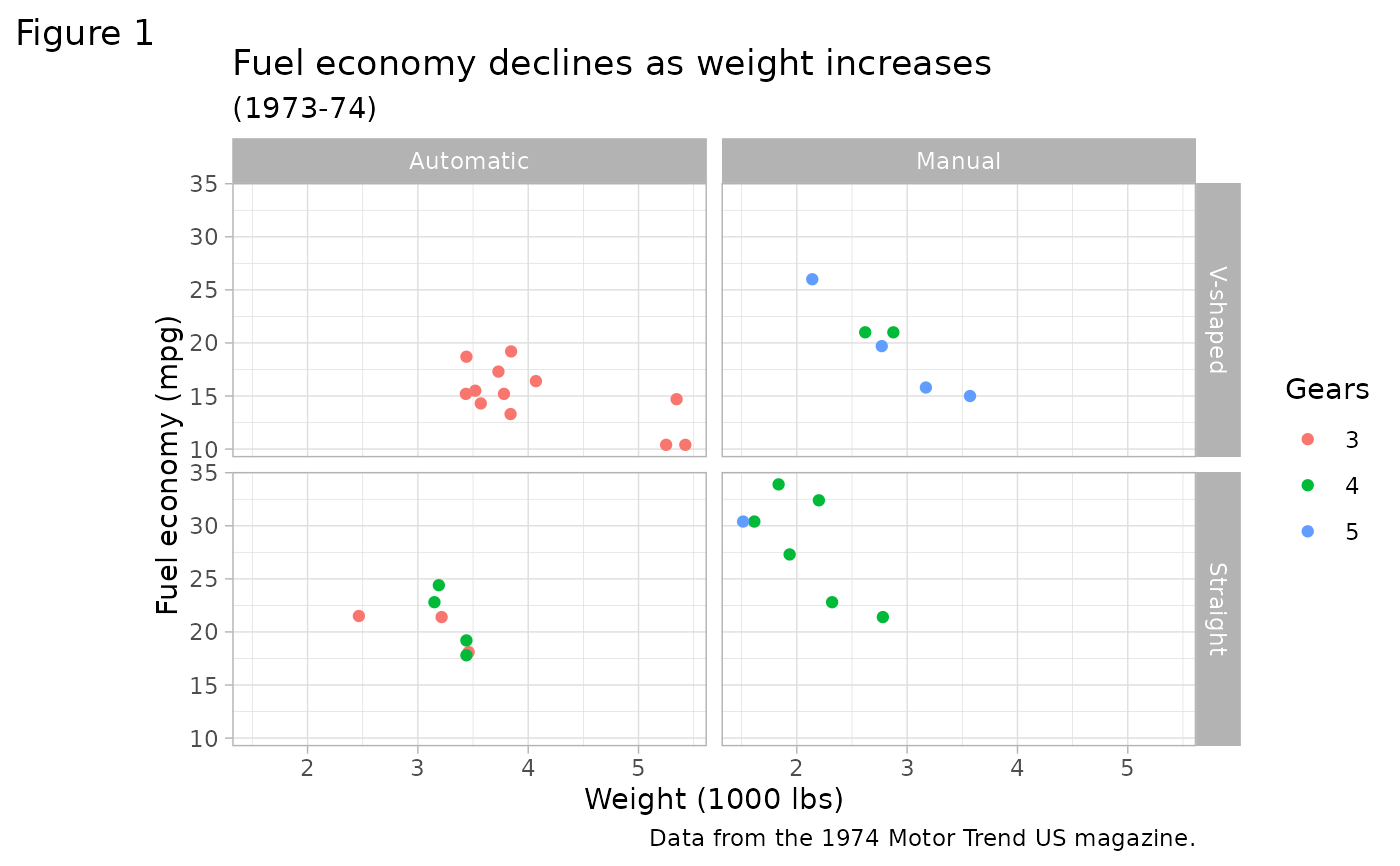p2 + theme_dark()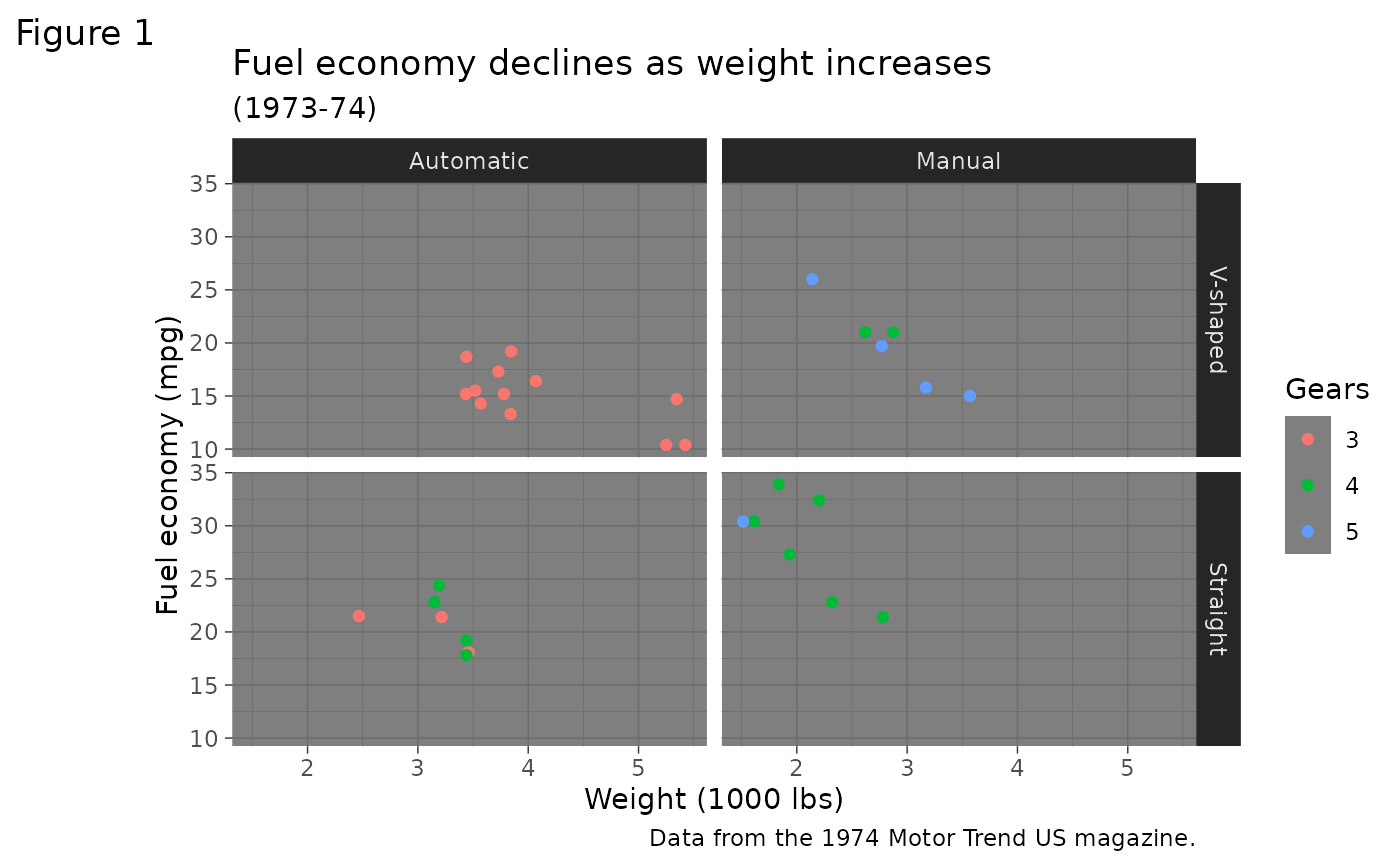p2 + theme_minimal()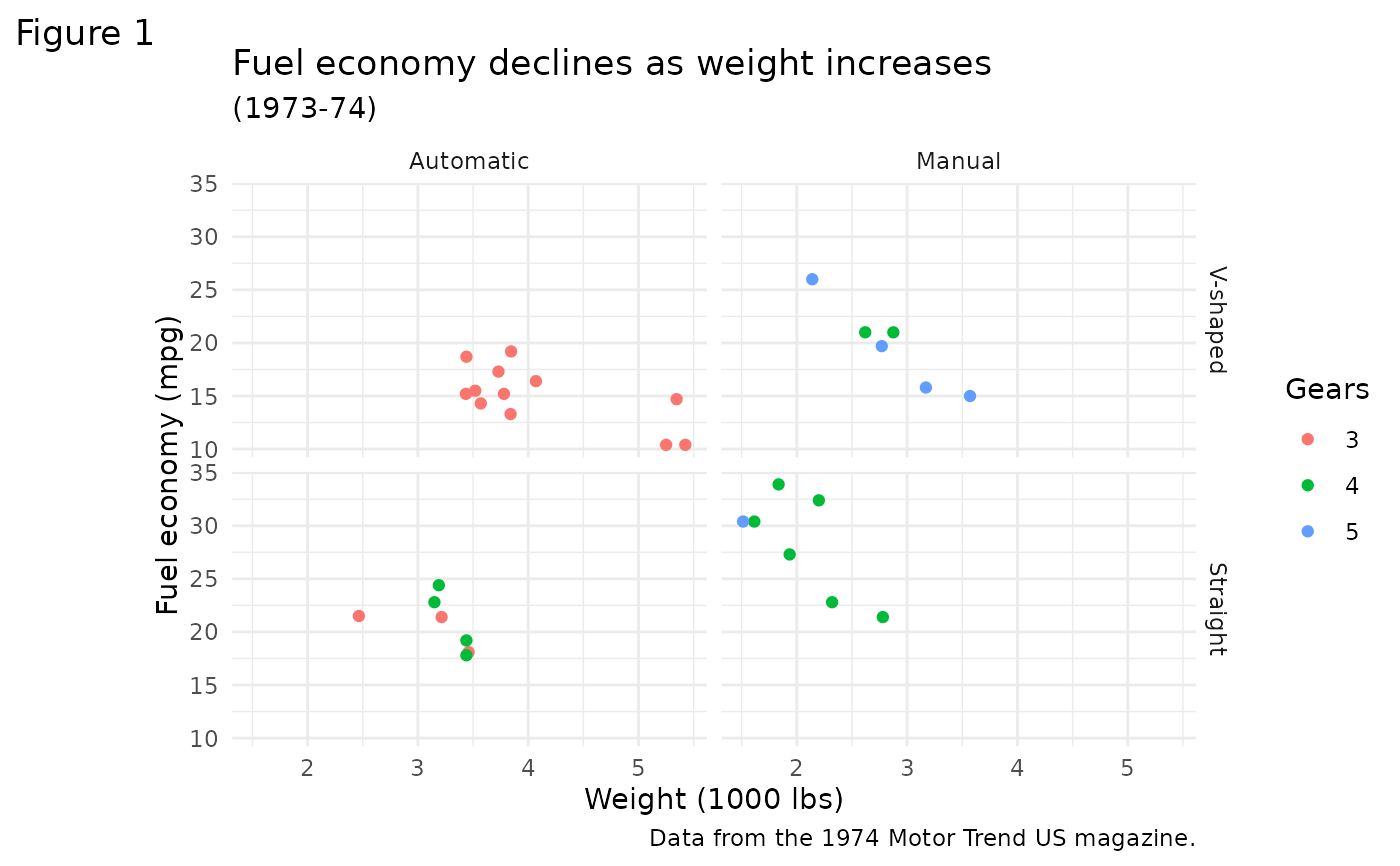p2 + theme_classic()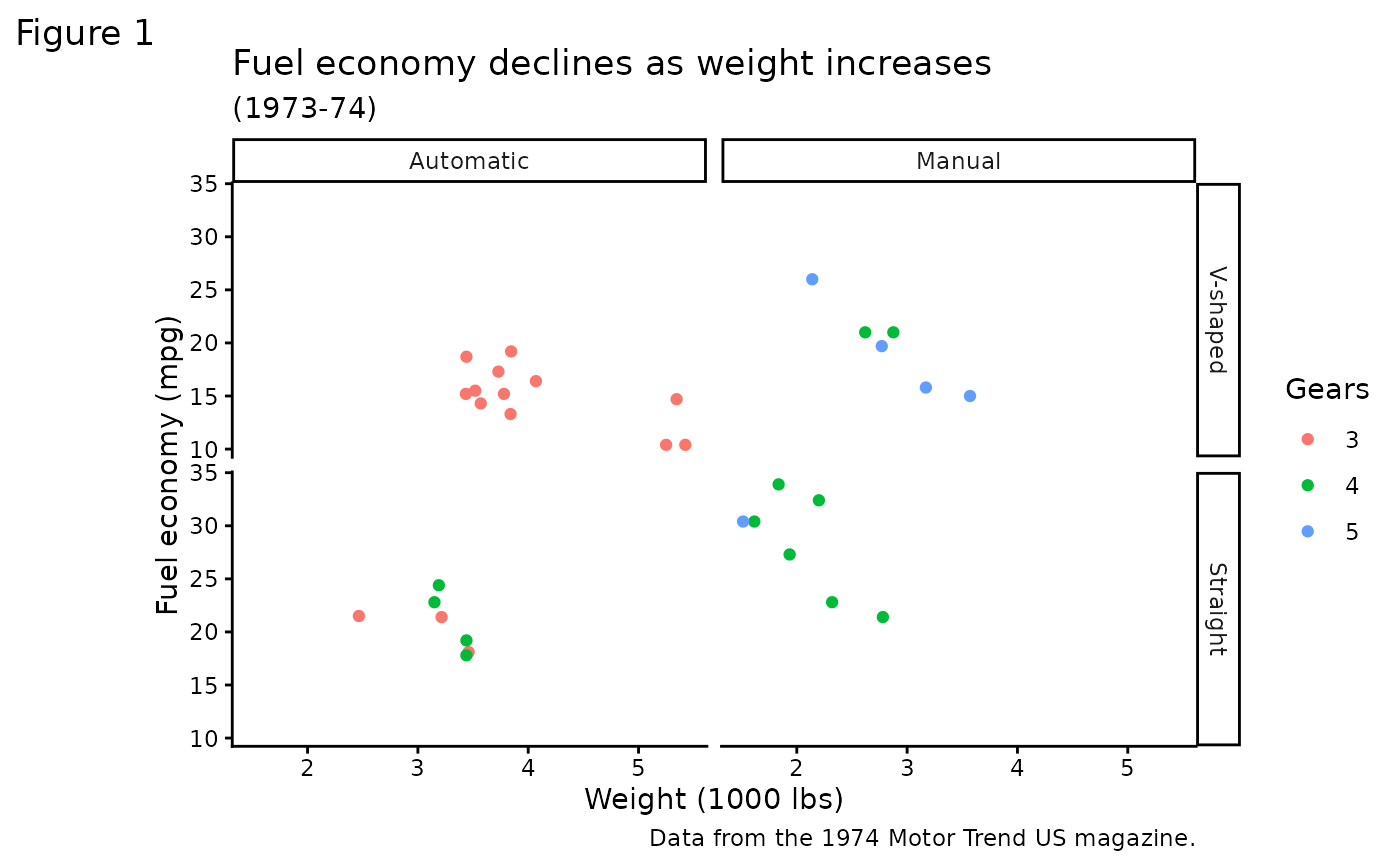p2 + theme_void()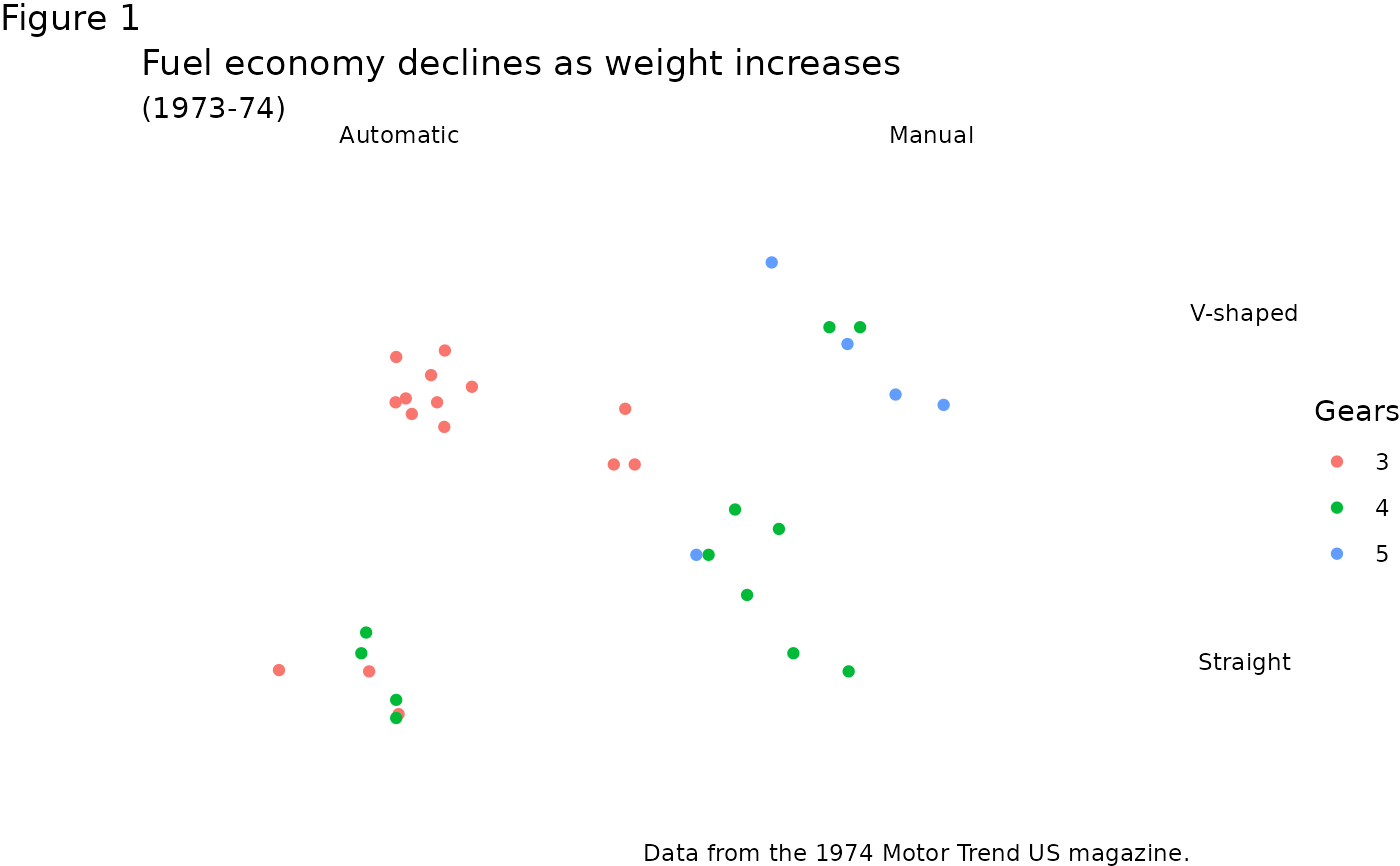# }